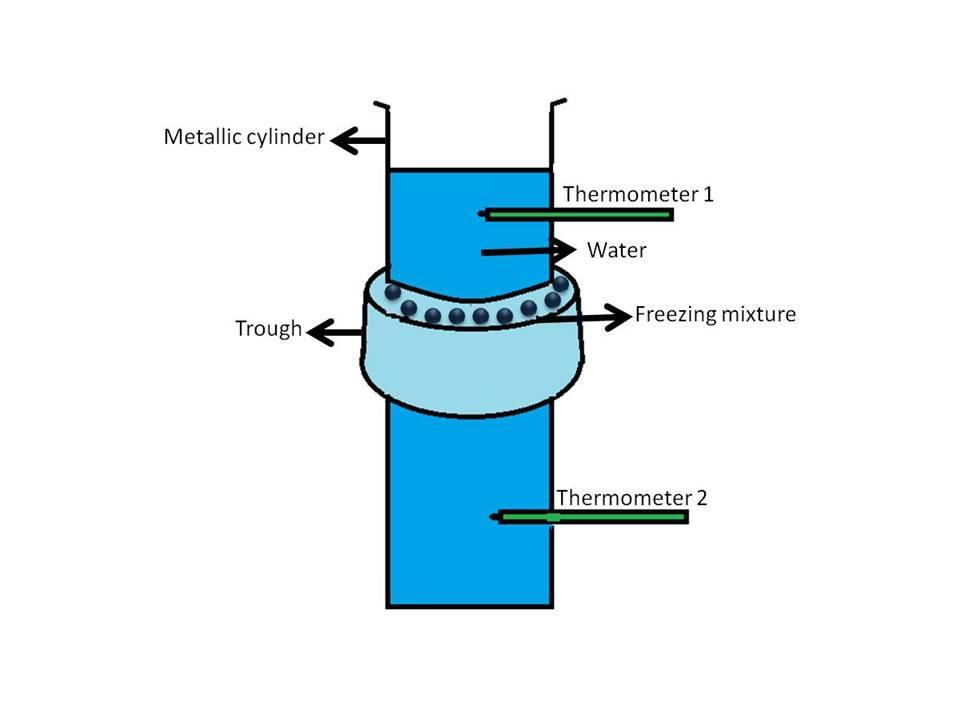Courses
Courses for Kids
Free study material
Free LIVE classes
MoreLIVE
Join Vedantu’s FREE Mastercalss

# Explain how Hope’s apparatus can be used to demonstrate the anomalous behavior of water?Verified
267.8k+ views
Hint: Hope’s apparatus is a vertical vessel containing water surrounded around the middle with a trough which is filled with ice. Two thermometers are placed above and below the trough to measure the temperature of the water as shown below:Complete answer:We all know that the density of solids is greater than liquids so it is expected that when a liquid becomes solid its density increases and it contracts. But water on cooling shows contraction till $4^oC$ and after that expands and converts into ice which has a lower density than water (clear from the fact that ice floats on water). This anomalous (different) behavior of water can be demonstrated by the use of an instrument called Hope’s apparatus. In this experiment the temperature of the water-filled in a cylinder vessel is varied. It is conducted in two sets:
$1.$ In the first set, the water is maintained at a temperature higher than $4^oC$, and the cylindrical vessel is filled with this water. The reading of the two thermometers is noted (it is observed to be the same in the beginning). Then freezing mixture containing ice and salt id added to the trough and after some time (say $30$ minutes) temperature is noted. In this case, the temperature of the water in the lower part of the vessel below the trough is found to be less as compared to the upper part. It can be explained by keeping in mind that the addition of freezing mixture decreased the temperature of the water and due to an increase in density (above $4^oC$) the water at lower temperature sinks down resulting in a lowering of the temperature of the thermometer $2$ as compared to the temperature of thermometer $1$.
$2.$ In the second set, the water is maintained to a temperature near to $4^oC$ and filled in the cylinder. After the addition of the freezing mixture, the temperature of water drops below $4^oC$ and it begins to expand thus comes to the upper part (remember, ice floats on water). Thus, the temperature of the upper part of the vessel decreases resulting in a lowering of the temperature of the thermometer $1$ as compared to the temperature of the thermometer $2$.

Note:
During the experiment, the temperature of the water should vary between $0^oC$ to higher than $4^oC$ otherwise the change will not be observed. Both water to be filled in the vessel and the apparatus itself is maintained at the same temperature.

Last updated date: 25th Sep 2023
Total views: 267.8k
Views today: 6.67k